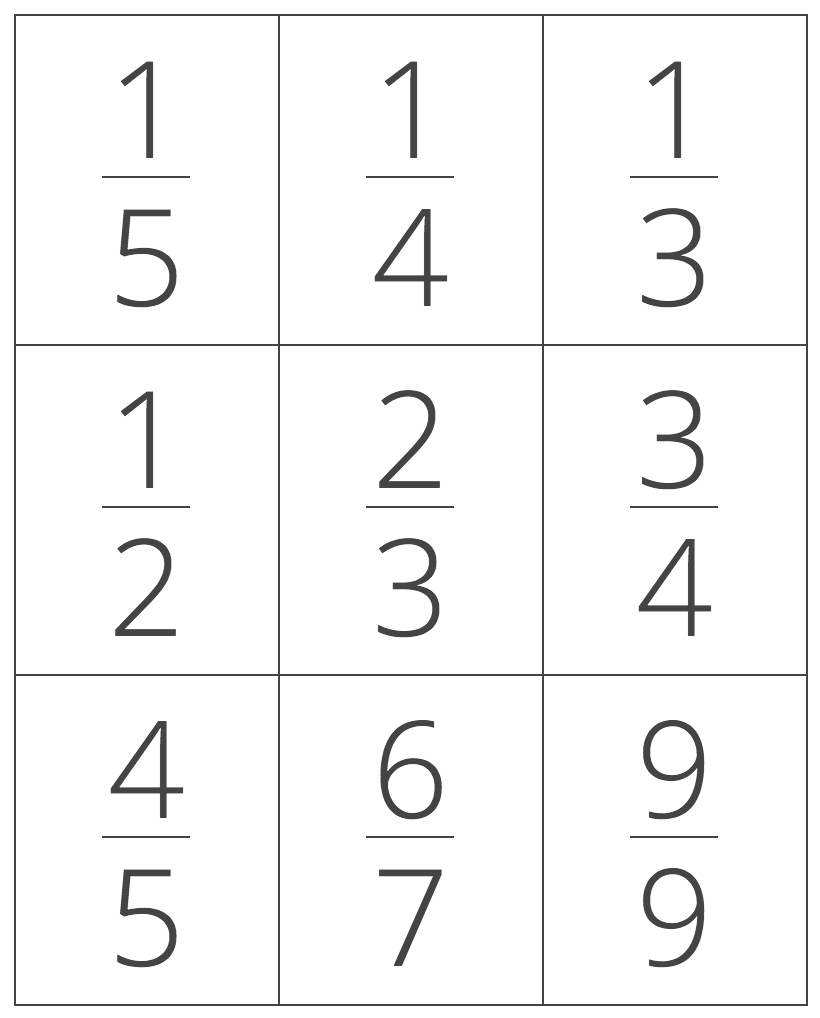# Least to Greatest Calculator

Sort a list of fractions, decimals, and percent numbers from least to greatest or greatest to least by entering the unsorted list and selecting the sort order. Follow along to see the steps used to sort the list.

Hint: separate numbers using a comma (,)
Sort By:

## Numbers Sorted Least to Greatest:

3 / 5 < 75% < 2.25 < 2 5 / 8

### Steps to Sort Numbers

Step One: convert each number to the equivalent decimal value

 3/5 2 5/8 2.25 75% 0.6 2.625 2.25 0.75

Step Two: sort the list of decimals from least to greatest

 3/5 75% 2.25 2 5/8 0.6 < 0.75 < 2.25 < 2.625

Step Three: rewrite the list using the original values

3 / 5 < 75% < 2.25 < 2 5 / 8
Learn how we calculated this below

## How to Sort Numbers from Least to Greatest

You can sort a list of fractions, decimals, whole numbers, and percentage values from least to greatest or vice-versa by following a few easy steps.

1. Convert each number to a decimal value
2. Compare each number and put in order
3. Add a comparison symbol between each number
4. Rewrite each number in its original form

Step One:
The first step to putting a set of numbers in order is to change them all into the same form. The easiest form to compare is decimal form, so convert fractions to decimal and percentages to decimal.

Step Two:
Then, compare each number and put smaller numbers on the left and larger numbers on the right. If you want to sort from greatest to least, then put larger numbers on the left and smaller numbers on the right.

Step Three:
After you arrange the numbers in order, add a comparison symbol between each one to compare the numbers. (see the “What are Comparison Symbols?” section below).

Step Four:
The final step is to rewrite each number in its original form. Convert decimals back to fractions or back to percentages if needed.

### What are Comparison Symbols?

A comparison symbol, or comparison operator, is a mathematical symbol used to compare two numbers. The most commonly used comparison symbols when comparing two numbers are less than (<), greater than (>), and equal (=).

When one number is smaller than another, the less than symbol is used like this:

1 < 2

And, when one number is larger than another, the greater than symbol is used:

2 > 1

### Ascending vs. Descending Order

After converting to decimal, sort the list by comparing each decimal value. There are a few different methods for sorting: ascending order or descending order.

Numbers in ascending order are sorted from least to greatest, while numbers in descending order are sorted from greatest to least.

To change the sort order from ascending to descending, simply flip the less than (<) or greater than (>) symbol that’s between each number to the opposite direction.

## How to Sort Fractions

Ordering a set of fractions might seem intimidating, but it’s possible to sort the list using a few different methods.### Method One: Order Fractions by Converting to the Same Denominator

The first method allows for sorting a list of fractions in just a few simple steps.

First, convert any mixed numbers to a fraction. If you are comparing whole numbers, then convert the whole number to a fraction by placing the number over 1.

The next step is to find the least common denominator for all of the fractions. This will be the same as the least common multiple (LCM) of the denominators. If you’re not sure how to do this, check out our LCD calculator.

After you have found the least common denominator, convert each fraction to the equivalent fraction with that denominator. To do this, divide the least common denominator by the fraction’s denominator.

Then multiply this number by the fraction’s numerator to find the converted numerator. Place the converted numerator over the least common denominator to get the converted fraction.

It is now possible to sort the converted fractions by simply ordering the numerators from small to large.

### Method Two: Order Fractions by Converting to Decimal

A more straightforward method of ordering fractions is to convert each fraction to a decimal, then order them.

After all of the fractions are in decimal form, order the decimals from small to large or large to small, depending on if ascending or descending order is desired.